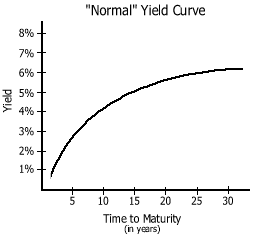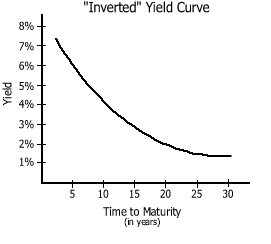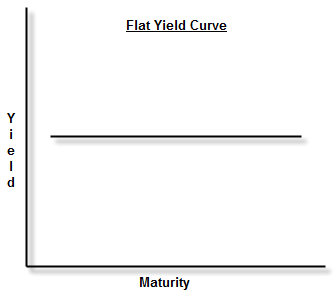# Yield Curve

The yield curve shows the relationship between the yield (interest rate) and the maturity period of a bond. It graphically represents the rate of return of a particular bond against its maturity period. Investors use yield curves to analyze and compare between their investments alternatives. It gives a clear understanding to the investor as to how much of yield that he is going to get depending on the no of years to the maturity. Investors use yield curves to speculate the future performance of the economy/firm based on short term and long term interest rates.

Relationship between Interest Rate and the Yield Curve.

There are 03 types of yield curves can be identified based on short term/long term interest rates and their shape. These 03 types can be identified as:

01.  Positive Yield curve

02.  Inverse yield curve

03.  Flat yield curve

01.  Positive Yield Curve

In other words this yield curve is called a normal yield curve where the long term interest rates are higher than the short term interest rates. This is an upward sloping curve and an illustration of a normal yield curve is shown below.The causes for this curve can be identified as investor’s speculations of economic growth. In other words economic growth creates higher inflation and to compensate the higher inflation investor would demand higher interest rates in long term.

02.  Inverse Yield Curve

This is called a negative yield curve and this is a situation where the short term interest rates are higher than the long term interest rates. This is a downward sloping curve and an illustration of a inverse yield curve is shown below.The reason for this curve to be downward sloping is investor’s expectation of economic downturn. In other words when economy is under performs or experience a negative economic growth the inflation rates would fall. Since the inflation rates fall investors will not be demanding for higher interest rates. As results the long term interest rates would be lower than the short term interest rates.

03.  Flat Yield Curve

This is a situation where the interest rates are expected to be constant over the given period of time. But in practice this is a rare situation. This is a curve which is parallel to the X axis of the graph which is the maturity period. An illustration of a normal yield curve is shown below.The reason for this graph is identified as investors are not sure of the future economic situations hence they tend to demand a constant return irrespective of inflation.